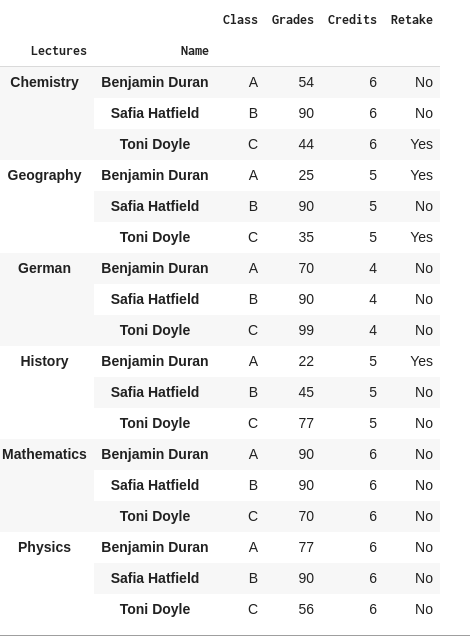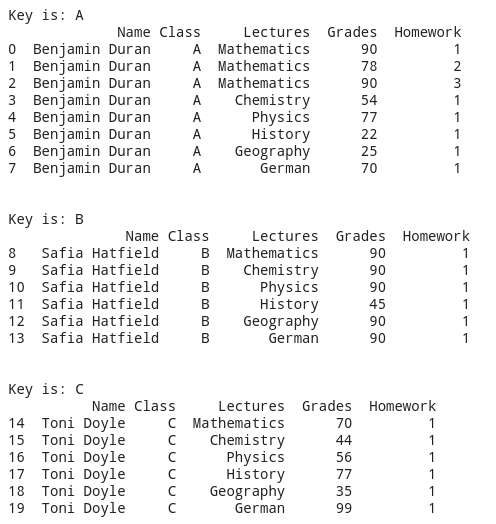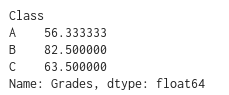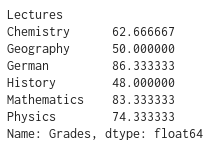Last Updated: November 24, 2021

# How to group data in Python using Pandas

Before we start: This Python tutorial is a part of our series of Python Package tutorials. The steps explained ahead are related to the sample project introduced here.

The Pandas groupby function lets you split data into groups based on some criteria. Pandas DataFrames can be split on either axis, ie., row or column.

To see how to group data in Python, let’s imagine ourselves as the director of a highschool. We can see how the students performed by comparing their grades for different classes or lectures, and perhaps give a raise to the teachers of those classes that performed well.

If we have a large CSV file containing all the grades for all the students for all their lectures, simply iterating through this DataFrame one by one and checking all the data would be too much work. Instead,  we can use Pandas’ groupby function to group the data into a Report_Card DataFrame we can more easily work with.

We’ll start with a multi-level grouping example, which uses more than one argument for the groupby function and returns an iterable groupby-object that we can work on:

`Report_Card.groupby(["Lectures","Name"]).first()`As can be seen from the image above we grouped by lecture and then by student name. This will make it easier to work on the statistics of the lectures for given students.

Now let’s look at the inner structure of a groupby object. We can iterate over the object created by the following code snippet:

```grouped_obj = Report_Card.groupby(["Class"])
for key, item in grouped_obj:
print("Key is: " + str(key))
print(str(item), "\n\n")```

This code snippet will result in 3 groupby objects with keys A, B and C, which are the values in the Class column of our DataFrame. The result is shown below.We can also use the function mean on the Grades column to calculate the average grade for each of the classes.

`Report_Card.groupby(["Class"])["Grades"].mean()`

The result of this line of code is as follows:But it wouldn’t be fair to base teacher performance on just class grade average (which in our contrived example only contains one student each), so let’s look at lecture grade averages, as well:

`Report_Card.groupby(["Lectures"])["Grades"].mean()`This code groups the Report_Card DataFrame on the Lectures column, and applies a mean function to the Grades column in order to return the average of the numerical values. We can now see at a glance the average grade for all students in each lecture, giving us a better impression of how well each of the teachers performed.

#### Next steps

Now that you know how to group data using Python’s Pandas library, let’s move on to other things you can do with Pandas:

## Get The Machine Learning Packages You Need – No Configuration Required

We’ve built the hard-to-build packages so you don’t have to waste time on configuration…get started right away!

### Some Popular ML Packages You Get Pre-compiled – With ActivePython

Machine Learning:

• TensorFlow (deep learning with neural networks)*
• scikit-learn (machine learning algorithms)
• keras (high-level neural networks API)

Data Science:

• pandas (data analysis)
• NumPy (multidimensional arrays)
• SciPy (algorithms to use with numpy)
• HDF5 (store & manipulate data)
• matplotlib (data visualization)

Security:

• cryptography (recipes and primitives)
• pyOpenSSL (python interface to OpenSSL)
• passlib and bcrypt (password hashing)
• requests-oauthlib (Oauth support)
• ecdsa (cryptographic signature)
• PyCryptodome (PyCrypto replacement)
• service_identity (prevents pyOpenSSL man-in-the-middle attacks)

With deep roots in open source, and as a founding member of the Python Foundation, ActiveState actively contributes to the Python community. We offer the convenience, security and support that your enterprise needs while being compatible with the open source distribution of Python.